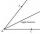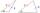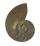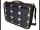Observer

The observer sees straight fence 100 m long in 30° view angle. From one end of the fence is 153 m.

How far is it from the another end of the fence?

Result

x =  179.9 m

Solution:Try calculation via our triangle calculator.

Leave us a comment of example and its solution (i.e. if it is still somewhat unclear...):Be the first to comment!To solve this verbal math problem are needed these knowledge from mathematics:

Do you have a linear equation or system of equations and looking for its solution? Or do you have quadratic equation? Do you want to convert length units? See also our trigonometric triangle calculator.

Next similar examples:Mast has 13 m long shadow on a slope rising from the mast foot in the direction of the shadow angle at angle 15°. Determine the height of the mast, if the sun above the horizon is at angle 33°. Use the law of sines.
2. Isosceles triangleWhat are the angles of an isosceles triangle ABC if its base is long a=5 m and has an arm b=4 m.
3. AnglesIn the triangle ABC, the ratio of angles is: a:b = 4: 5. The angle c is 36°. How big are the angles a, b?
4. AngleDraw angle |∠ ABC| = 130° and built its axis. What angle is between axis angle and arm of angle?
5. Trapezium ABCDIn the figure, ABDC is a trapezium in which AB || CD. line segments RN and LM are drawn parallel to AB such that AJ=JK=KP. If AB=0.5m and AP=BQ=1.8m, find the lengths of AC, BD, RN and LM. angle D=angle C=60
6. Trapezium internal anglesA trapezium where AB is parallel to CD, has angle A : angle D = 4 :5, angle B = 3x-15 and angle C = 4x+20. Find angle A, B, C and D.
7. Scalene triangleSolve the triangle: A = 50°, b = 13, c = 6
8. Reference angleFind the reference angle of each angle:
9. ABCDAC= 40cm , angle DAB=38 , angle DCB=58 , angle DBC=90 , DB is perpendicular on AC , find BD and AD
10. Theorem proveWe want to prove the sentence: If the natural number n is divisible by six, then n is divisible by three. From what assumption we started?
11. MedianThe median of the triangle LMN is away from vertex N 84 cm. Calculate the length of the median, which start at N.
12. What isWhat is the value of the smaller of a pair of numbers for which their sum is 78 and their division quotients are 0.3?
13. Two triangles SSATwo triangles can be formed with the given information. Use the Law of Sines to solve the triangles. A = 59°, a = 13, b = 14
14. Logarithmic equationSolve equation: log33(3x + 21) = 0
15. GainFind the gain whenever the output power is 21x the input power.
16. ReciprocalCalculate reciprocal of z=0.8-1.8i:
17. Linear imaginary equationGiven that ? "this is z star" Find the value of the complex number z.